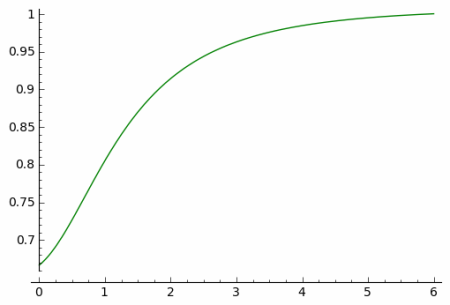# The single big jump principle

Suppose you’re playing a game where you take 10 steps of a random size. Here are two variations on the game. Which will give you a better chance of ending up far from where you started?

1. You take your steps one at a time, starting each new step from where the last one took you.
2. You return to the starting point after each step. After 10 turns, you go back to where your largest step took you.

Assume all steps are in the same direction. Then you’re always better off under the first rule. The sum of all your steps will be bigger than the maximum since the maximum is one of the terms in the sum.

However, depending on the probability distribution on your random steps, you may do almost as well under the second rule, taking the maximum of your steps.

If the distribution on your step size is fat-tailed (technically, subexponential) then the maximum and the sum have the same asymptotic distribution. That is, as x goes to infinity,

Pr( X1 + X2 + … + Xn > x ) ~ Pr( max(X1, X2, … , Xn) > x )

As x gets larger, the relative advantage of taking the sum rather than the maximum goes to zero. This is known as “the principle of the single big jump.” If you’re looking to make a lot of progress, adding up typical jumps isn’t likely to get you there. You need one big jump. Said another way, your total progress is about as good as the progress on your best shot.

Before you draw a life lesson from this, note that this is only true for fat-tailed distributions. It’s not true at all if your jumps have a thin-tailed distribution. But sometimes the payoffs in life’s games are fat-tailed.

What distributions are subexponential? Any fat-tailed distribution you’re likely to have heard of: Cauchy, Lévy, Weibull (with shape < 1), log-normal, etc.

To illustrate the single jump principle, let X1 and X2 be standard Cauchy random variables. The difference between Pr( X1 + X2 > x ) and Pr( max(X1 , X2) > x ) becomes impossible to see for x much bigger than 3.Here’s a plot of the ratio of the sum to the maximum.The ratio tends to 1 in the limit as predicted by the single big jump principle.

I chose to use a Cauchy distribution to simplify the calculations. (The sum of two Cauchy random variables is another Cauchy. See distribution relationships.) In this case the maximum is actually larger than the sum because the Cauchy can be positive or negative. But it is still the case that the two tails converge as x increases.

More on the single big jump here.

## 9 thoughts on “The single big jump principle”

1. Luis Carvalho

I think you mean max(X1, X2, …, Xn) instead of max(X1 + X2 + … + Xn)… A minor point: you’re assuming that the Xi, the steps, are *independent*, right?

2. What I take to be an earlier draft of the linked to book An Introduction to Heavy-tailed and Subexponential Distributions.

3. Luis: Thanks for pointing out the error. I corrected the post. And yes, I’m implicitly assuming independence.

4. Luis Carvalho

John: you forgot to change Pr(max(X1+X2)>x) to Pr(max(X1, X2)>x). :)

5. Thanks!

6. Thanks for the Sage Notebook. Great work.

7. You familiar with Sornette’s work?

8. No, I’m not.

9. I presume this DOES NOT constitute personal financial advice recommending that we abandon our retirement contributions in favor of weekly Lotto tickets. Unless our name is either Joan Gither or Mohan Srivastava.